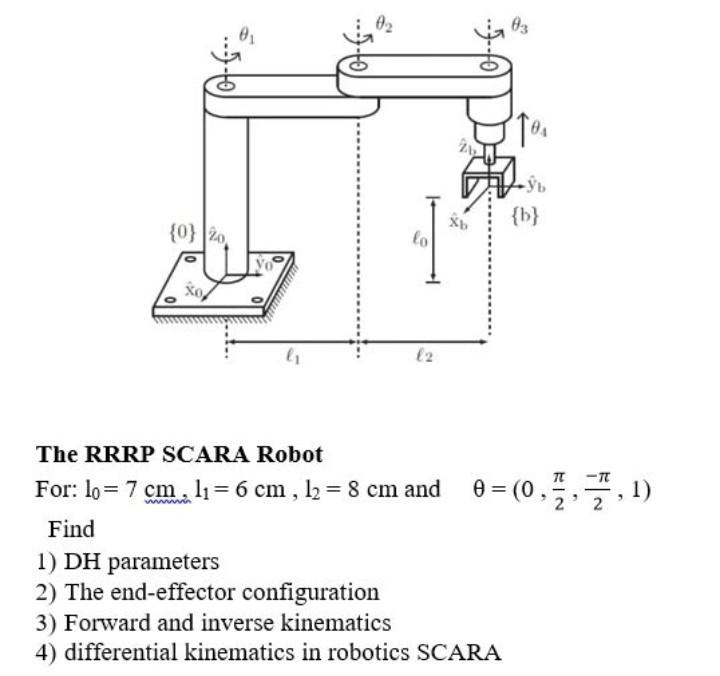Home / Expert Answers / Mechanical Engineering / i-need-a-solution-with-an-explanation-quickly-nbsp-the-rrrp-scara-robot-for-l-0-7-mathr-pa263

# (Solved): I need a solution with an explanation quickly   The RRRP SCARA Robot For: $$l_{0}=7 \mathr ... I need a solution with an explanation quicklyThe RRRP SCARA Robot For: \( l_{0}=7 \mathrm{~cm}, l_{1}=6 \mathrm{~cm}, l_{2}=8 \mathrm{~cm}$$ and $$\quad \theta=\left(0, \frac{\pi}{2}, \frac{-\pi}{2}, 1\right)$$ Find 1) $$\mathrm{DH}$$ parameters 2) The end-effector configuration 3) Forward and inverse kinematics 4) differential kinematics in robotics SCARA

We have an Answer from Expert